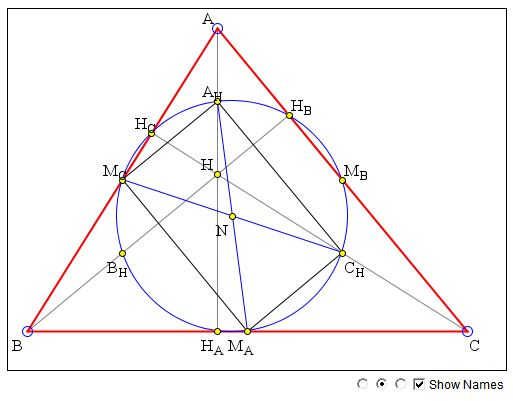# Nine Point Circle: What Is This About? A Mathematical Droodle

### If you are reading this, your browser is not set to run Java applets. Try IE11 or Safari and declare the site https://www.cut-the-knot.org as trusted in the Java setup.What if applet does not run?

ExplanationThe applet purports to suggest a proof for the existence of the 9-point circle. The proof was brought to my attention by Hubert Shutrick. Here is the statement of existence:

In ΔABC, the midpoints of the sides MA, MB, MC, the feet HA, HB, HC of the altitudes, and the midpoints AH, BH, CH of the segments connecting the orthocenter with the vertices, lie on a circle, known as the 9 point circle.

The proof is illustrated by the applet:First of all, MBMC is the midline in ΔABC so that it's parallel to BC and equals half of the latter. The same holds of BHCH which is a midline in ΔHBC. It follows that MBMCBHCH is a parallelogram. But more is true. MBCH is a midline in ΔAHC. In particular, this implies that MBCH is orthogonal to BC (as it is parallel to AH.) Hence the quadrilateral MBMCBHCH is a rectangle.

Observe that the diagonals of a rectangle (of a parallelogram in fact) cross at their midpoints. Let N be the center of the rectangle MBMCBHCH.

Rectangles MCMACHAH and MAMBAHBH are obtained in a similar way. Between them, the three rectangles share three diagonals: MAAH, MBBH, MCCH and therefore have a common center. This shows that 6 points - MA, MB, MC, AH, BH, CH lie on a circle with center N.

Furthermore, in ΔAHHAMA the angle at HA is right whereas the hypotenuse AHMA serves as a diameter of the just found circle. It follows that HA also lies on that circle, and similar argument applies to the feet of the remaining altitudes, HB and HC.

(Note: Hubert later suggested a shortcut. Once it was established that MBMCBHCH is cyclic, then the right angles give that HC and HB also lie on the circle. The similar circle defined by MCMACHAHHCHA has the points MC, CH, HC in common with the previous circle so they are the same. Another simple argument can be found elsewhere at the site.)### Nine Point Circle